# NCERT Exemplar Class 12 Physics Solutions for Chapter 10 - Wave Optics

NCERT Class 12 Physics Exemplar Solutions for Chapter 10 Wave Optics are provided here for Class 12 science students to ace their exams. This NCERT Class 12 Physics Exemplar Solutions for Chapter 10 provides you with answers to the NCERT exemplar problems Class 12 Physics PDF, along with extra questions prepared by subject experts, sample papers and previous years’ question papers.

This solution to Wave Optics Class 12 problems helps students to understand important numerical problems in the chapter Wave Optics. Students are advised to solve the Exemplar questions PDF to grasp the topics to score good marks in CBSE Class 12 examinations and graduate entrance examinations.

## Download the PDF of the NCERT Exemplar Class 12 Physics Chapter 10 Wave Optics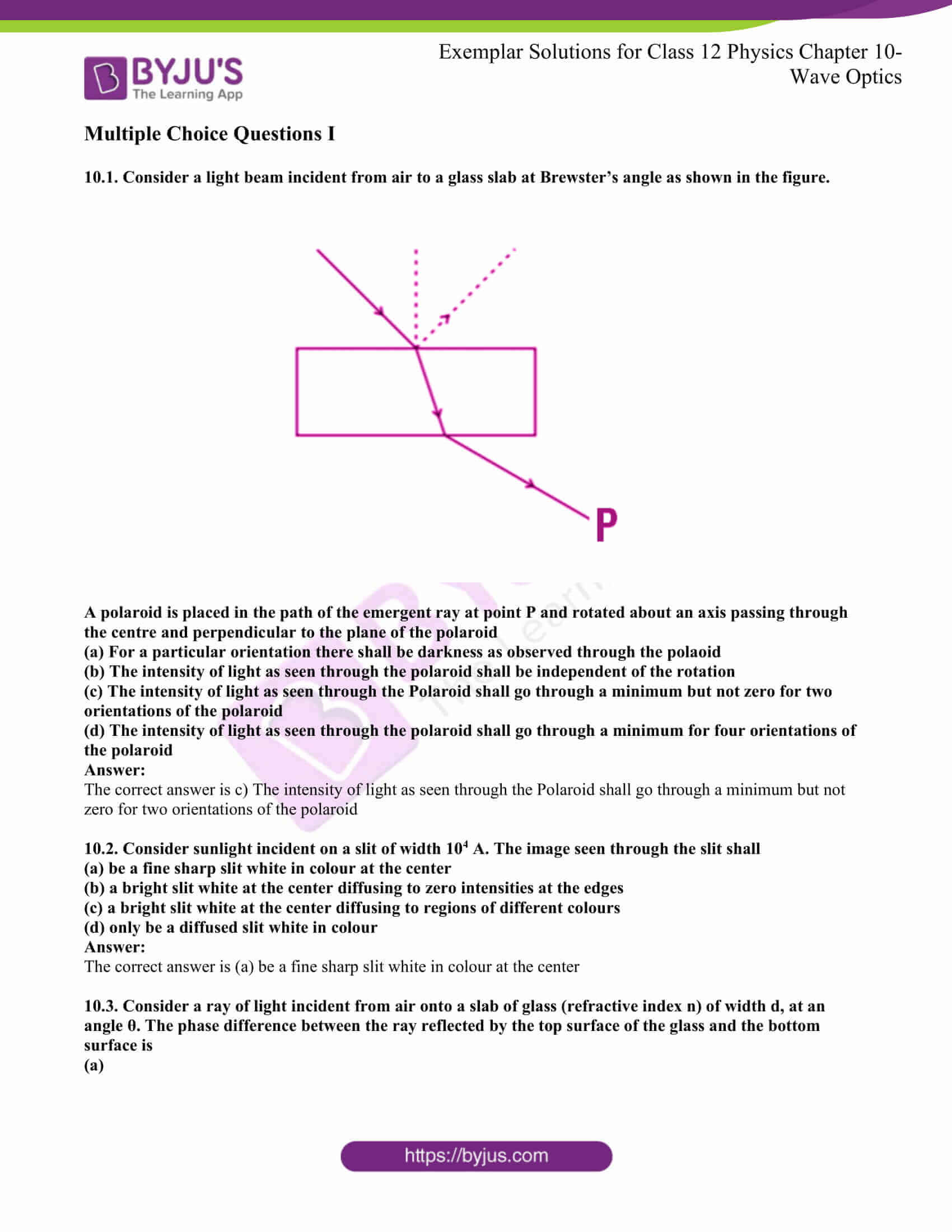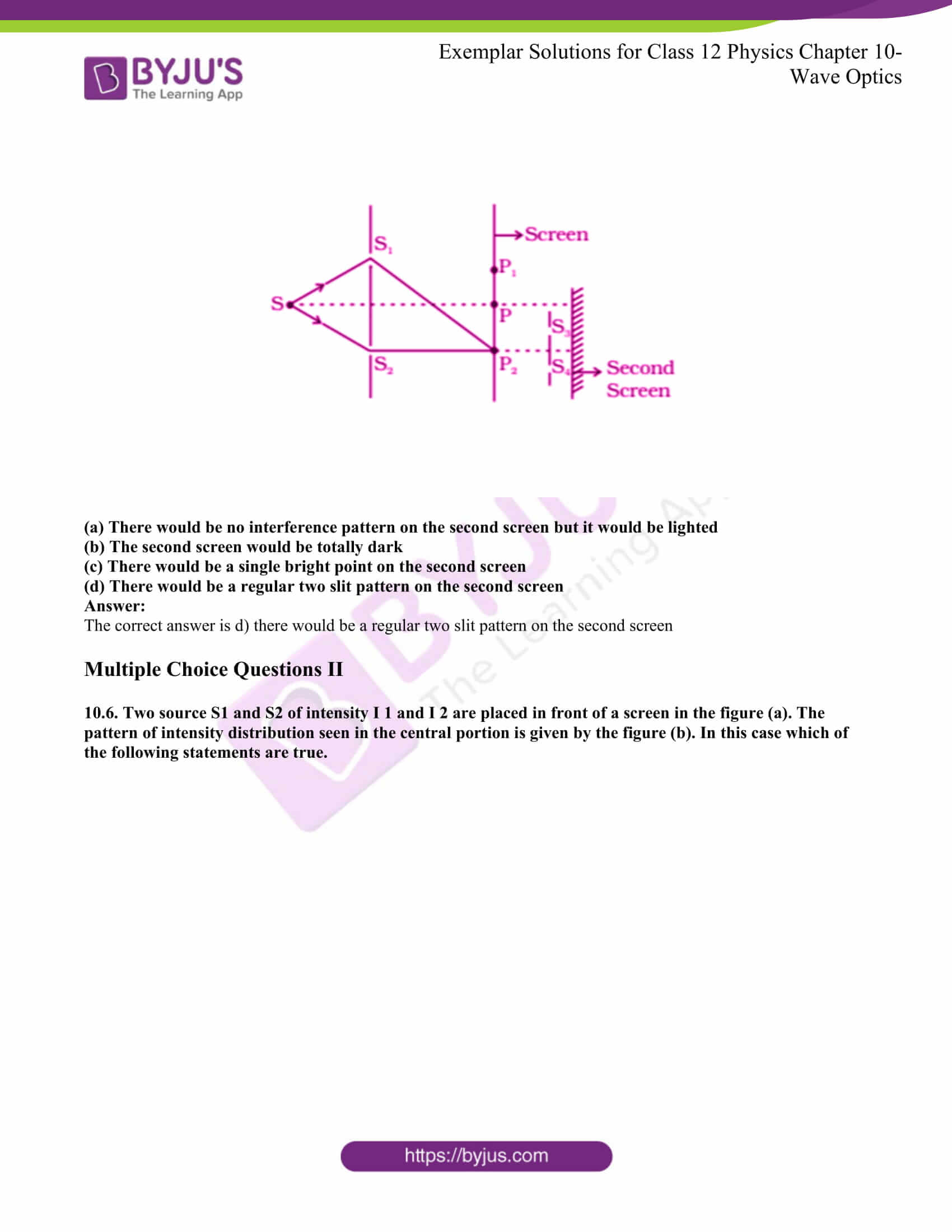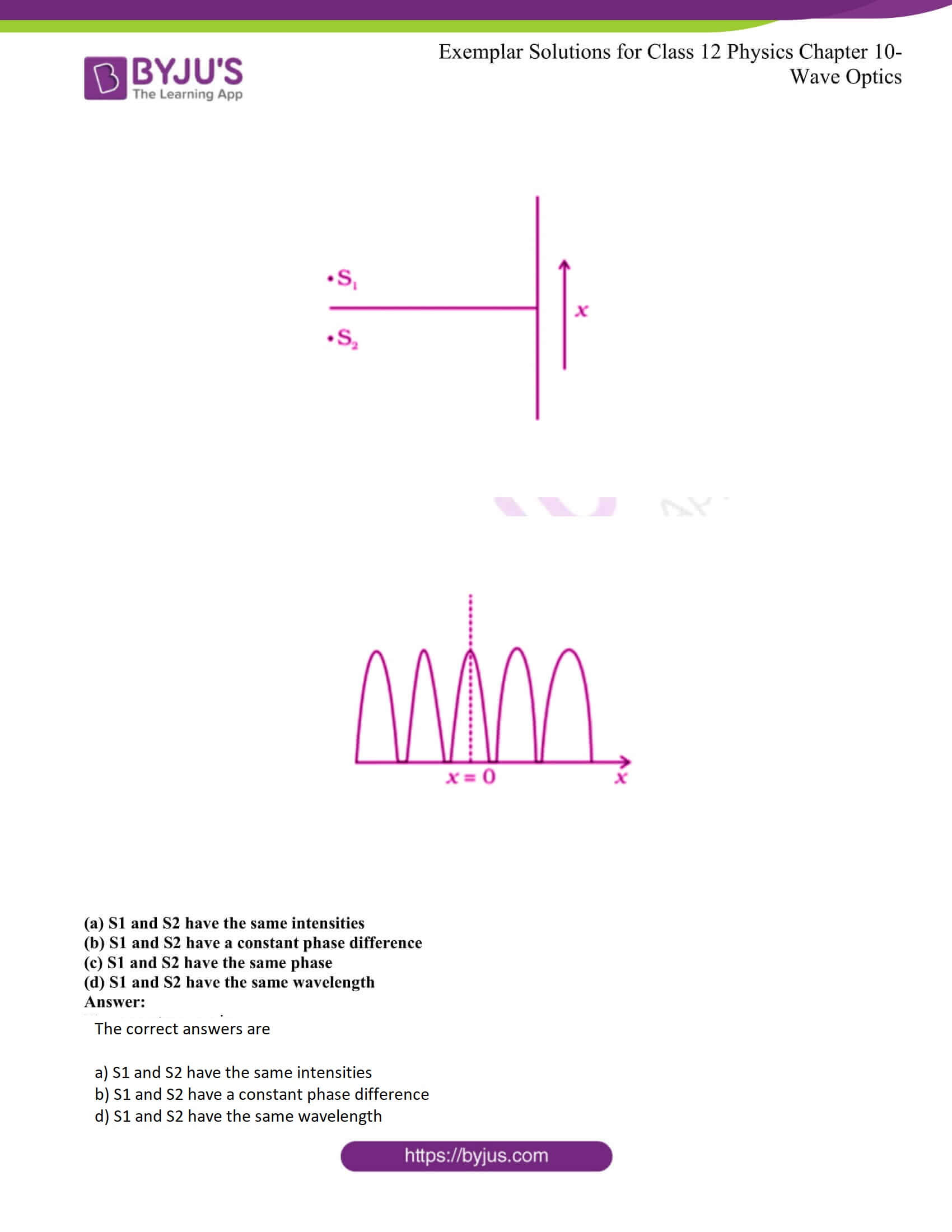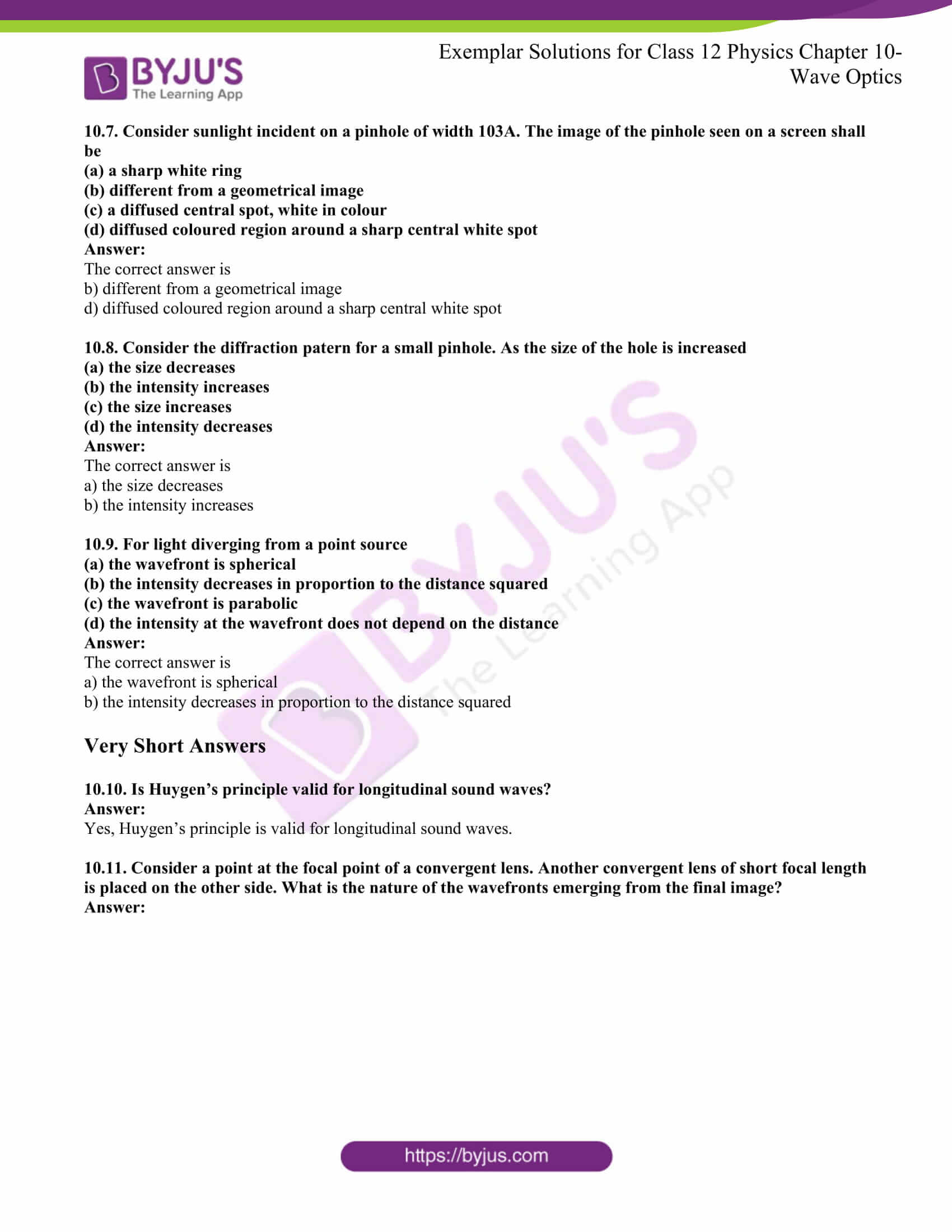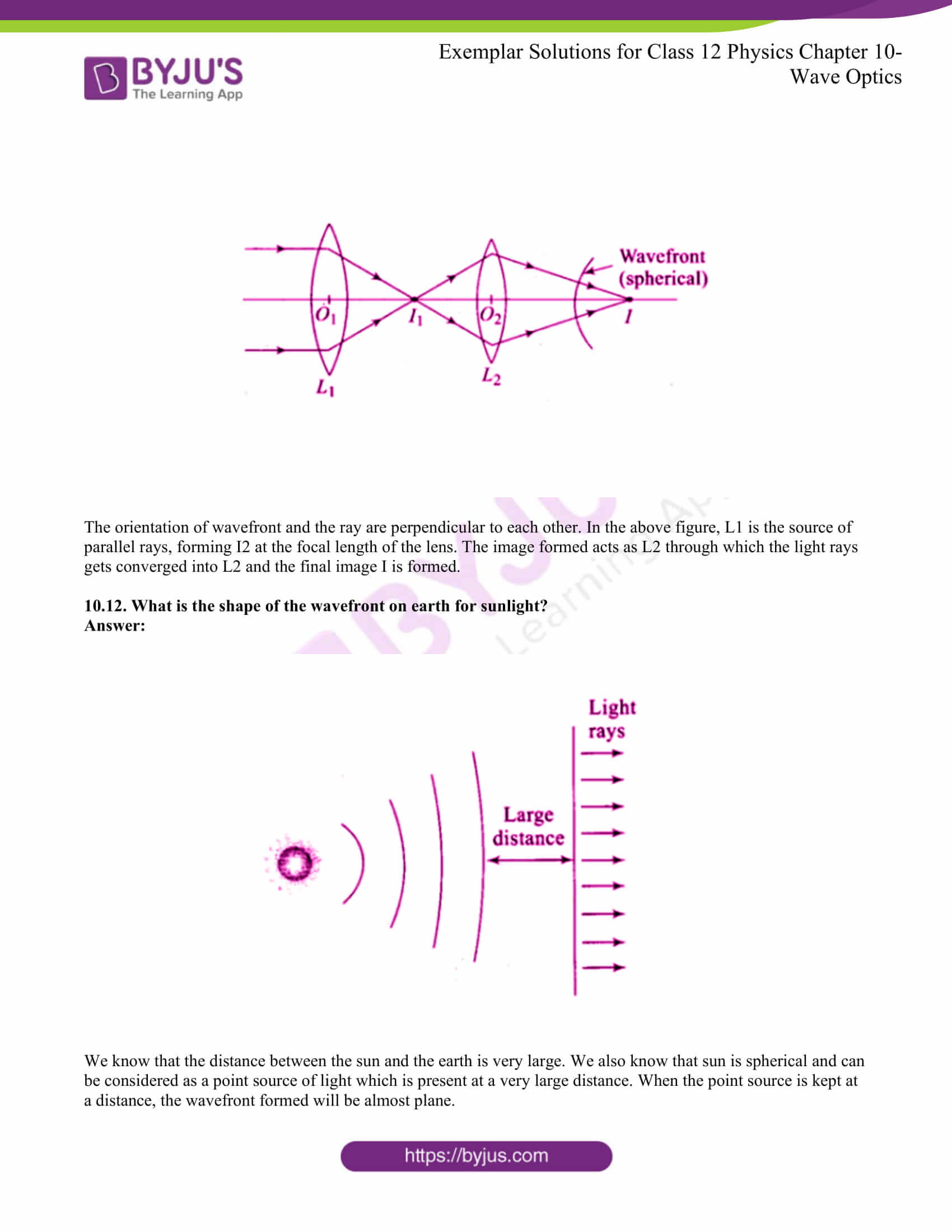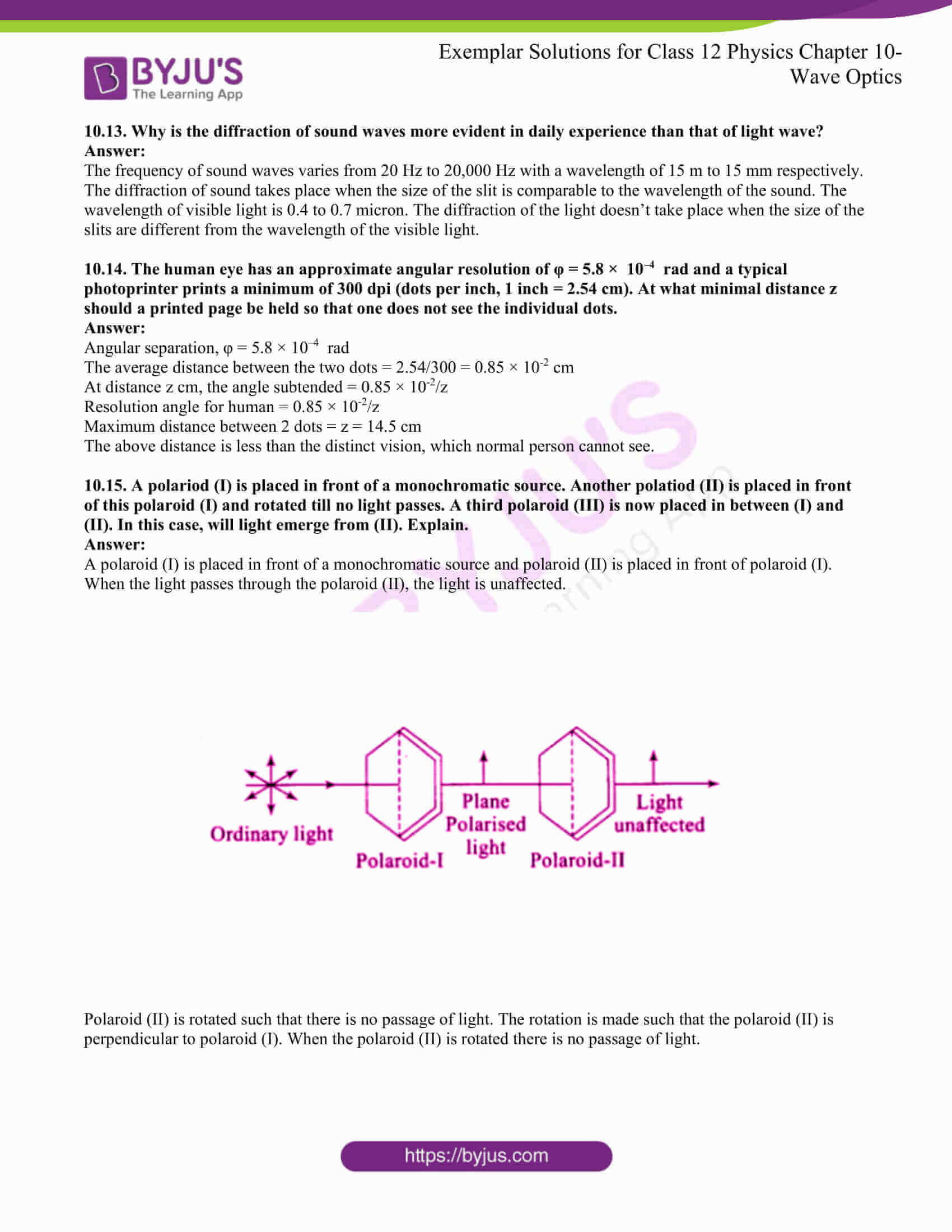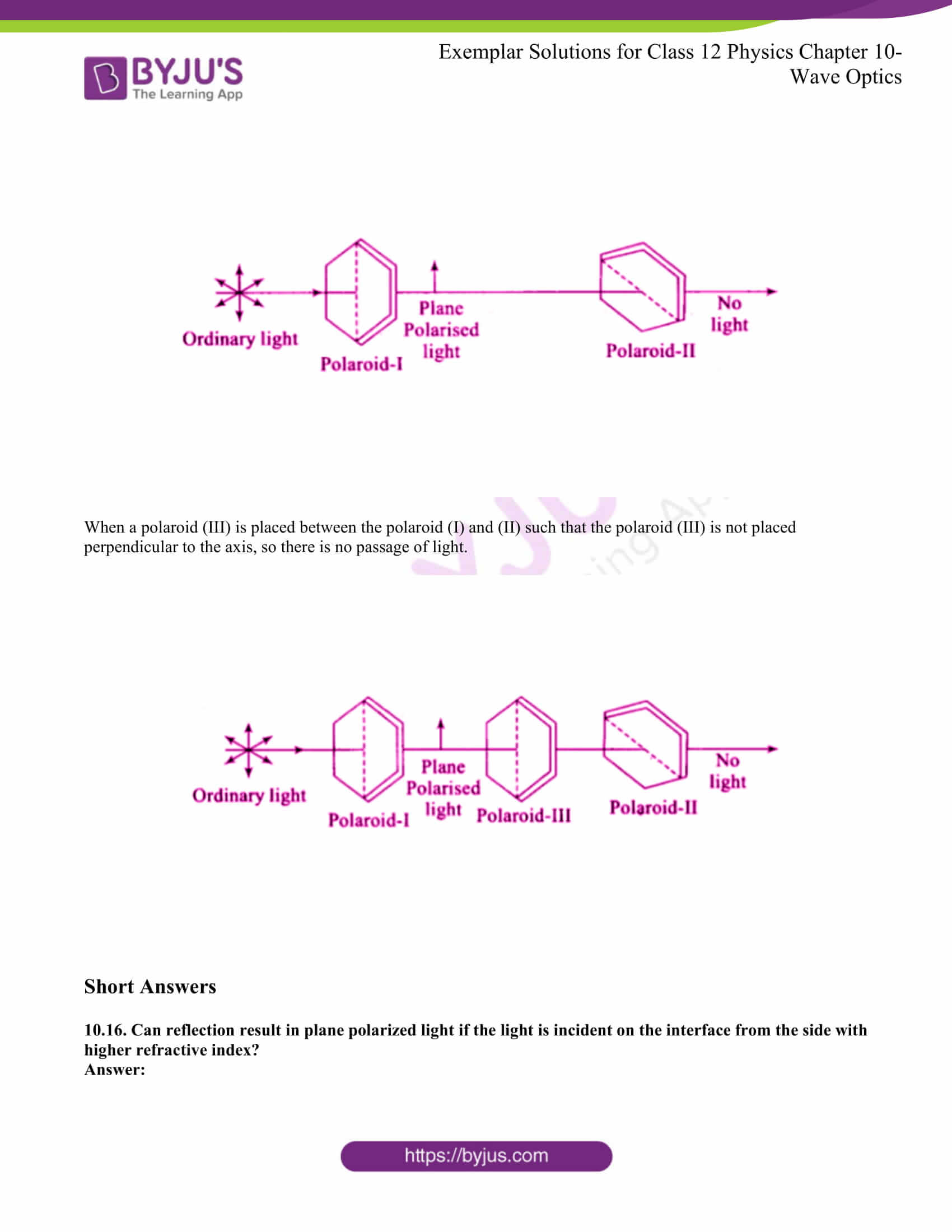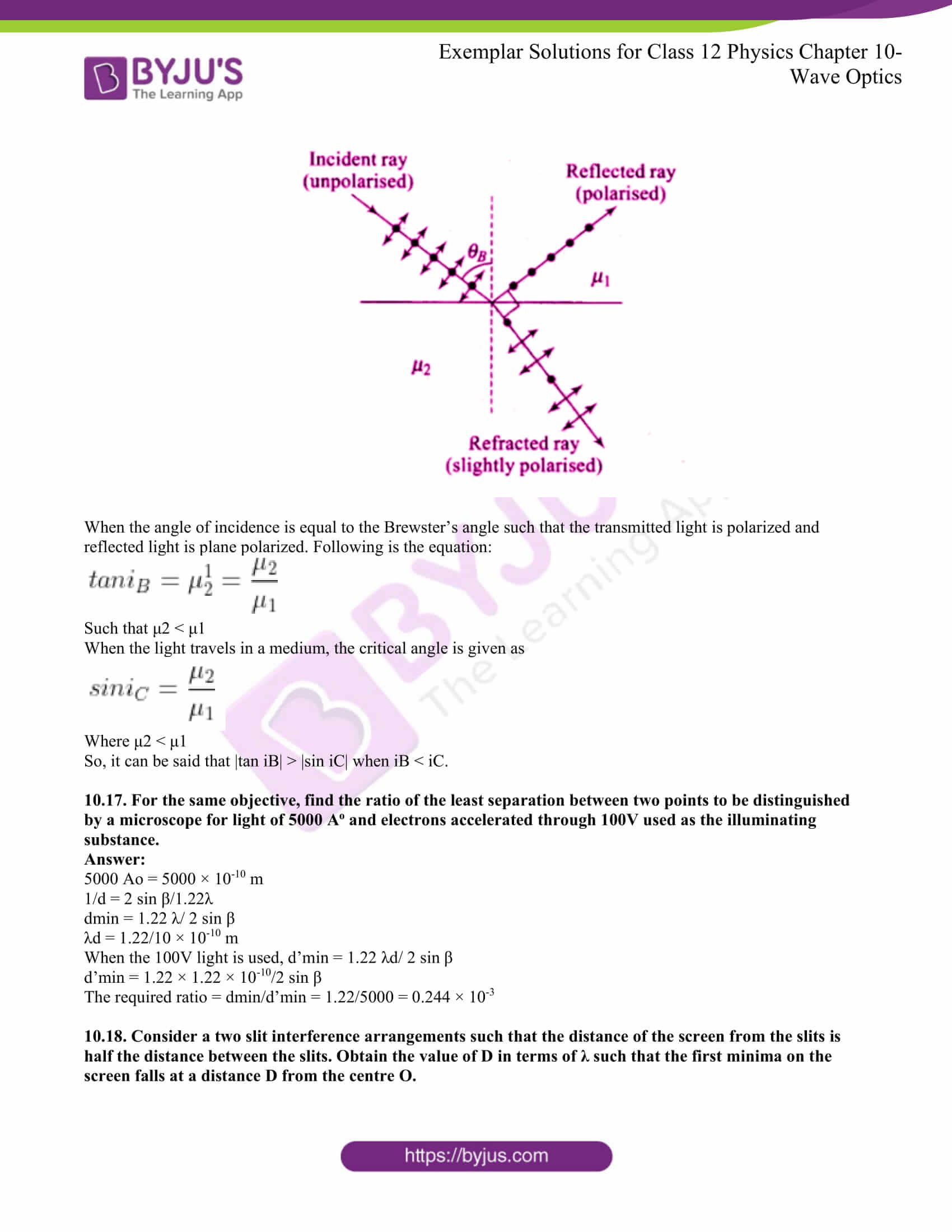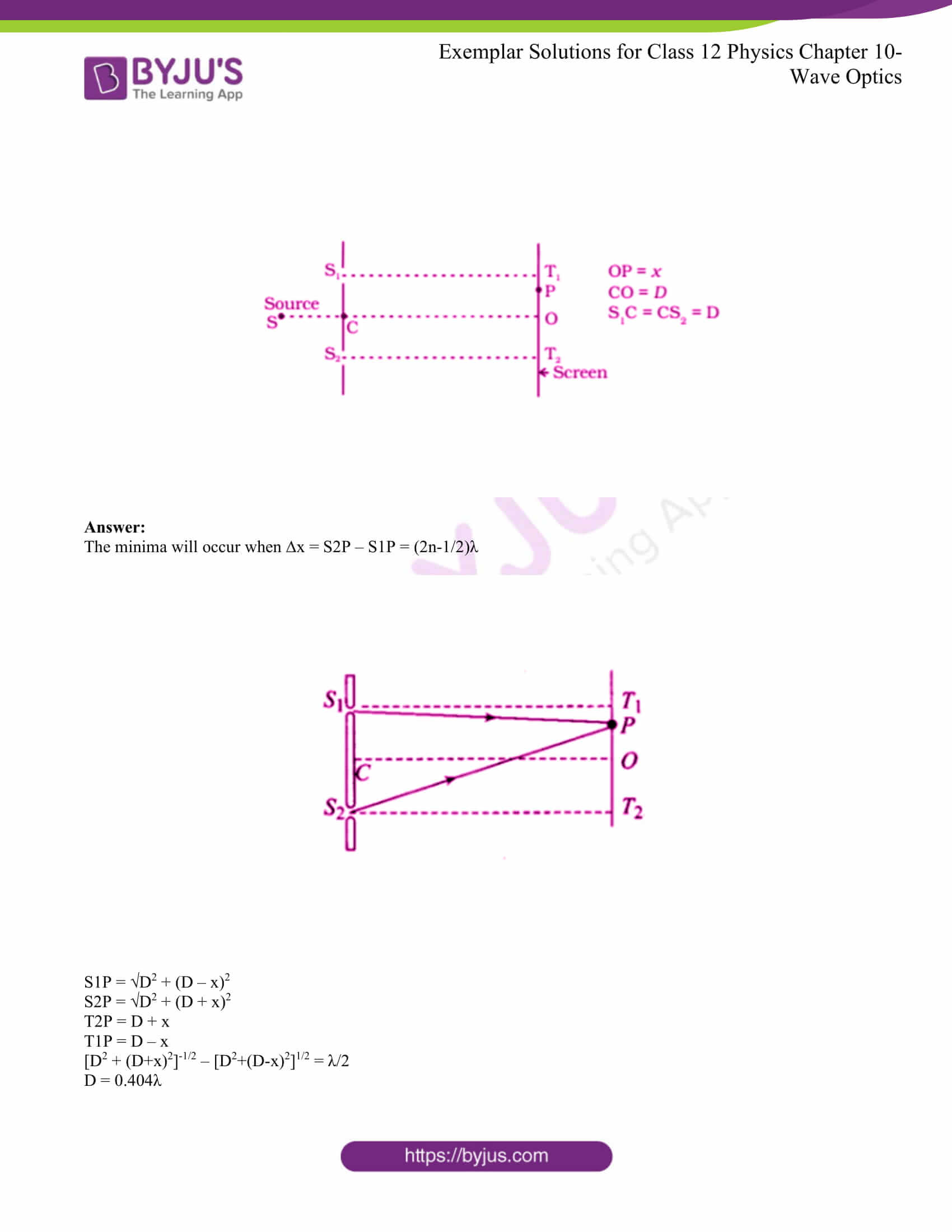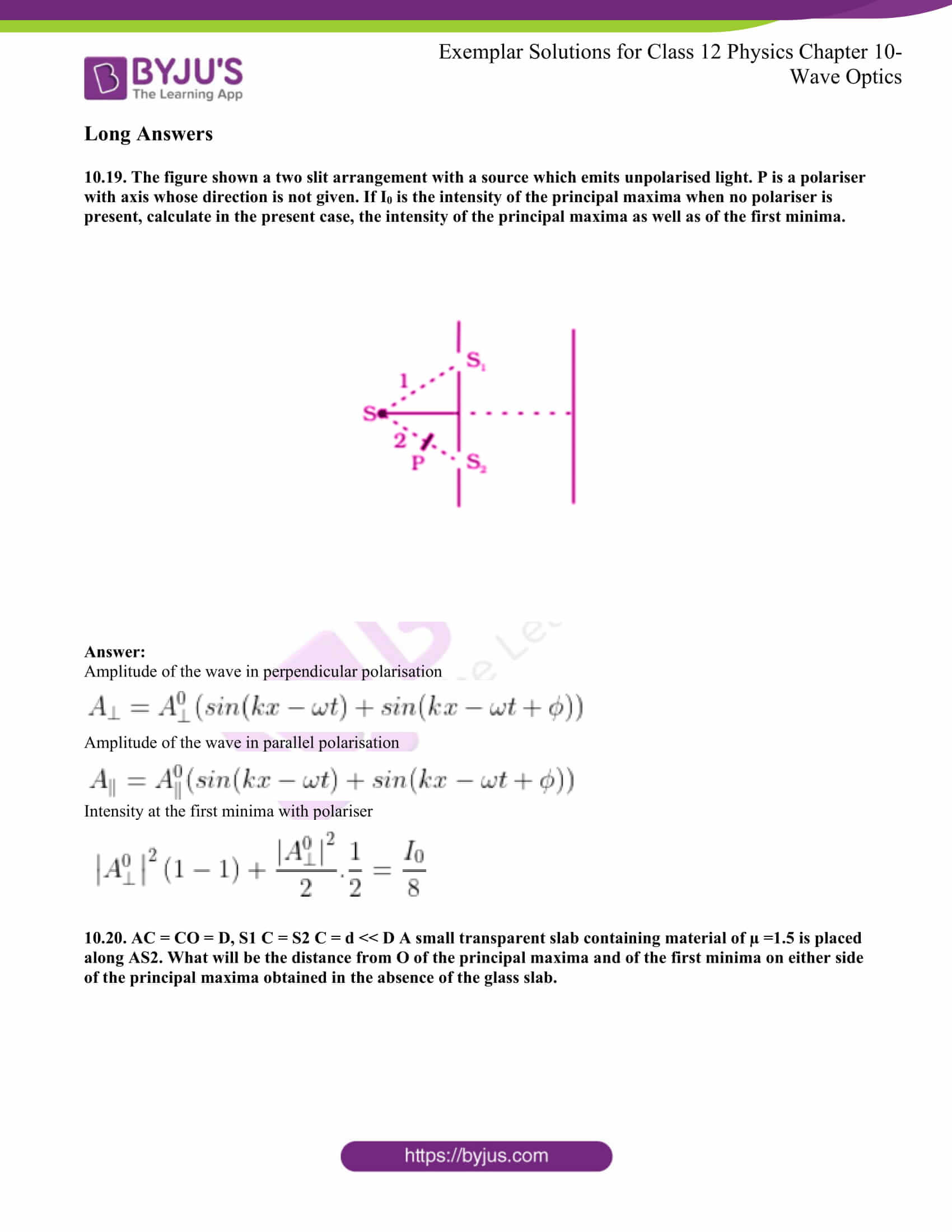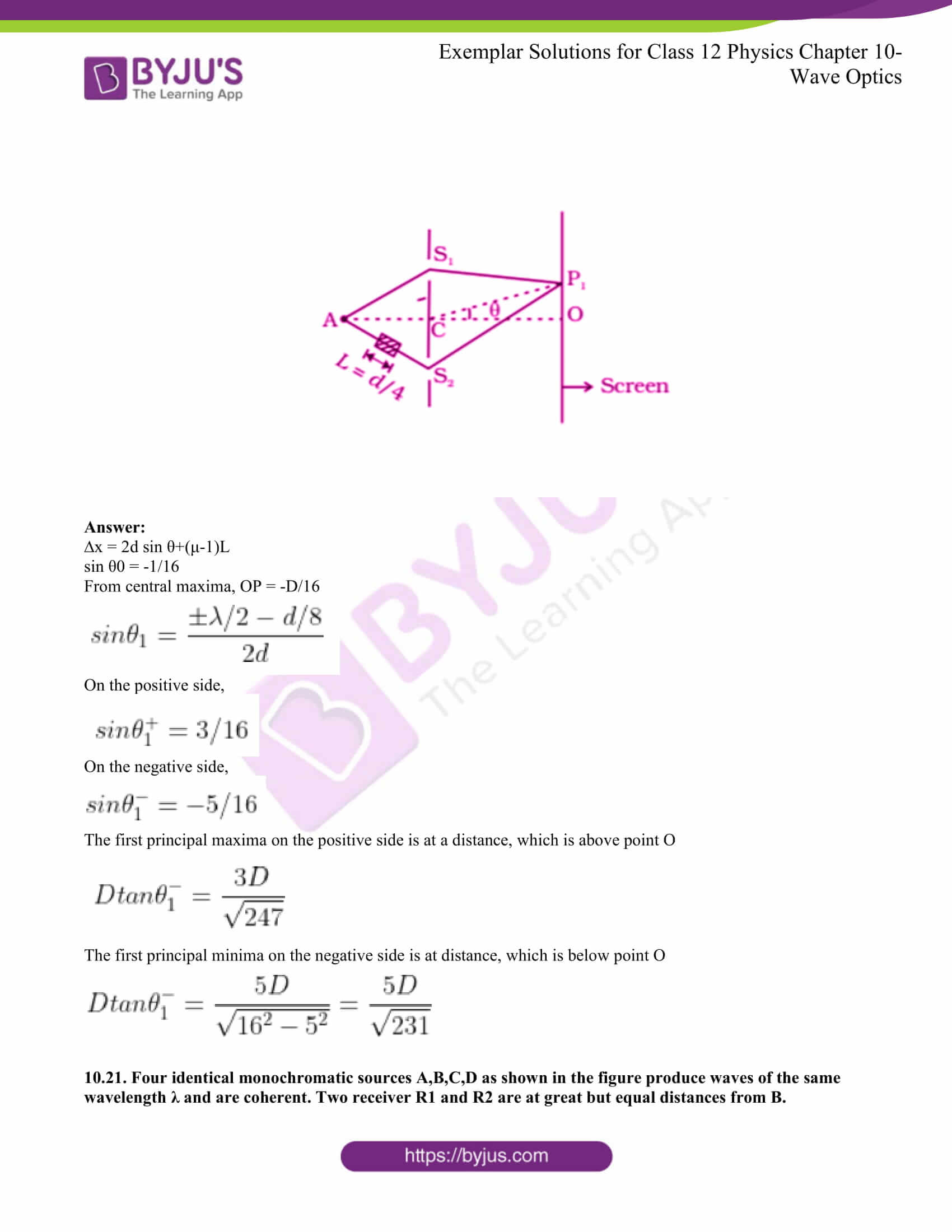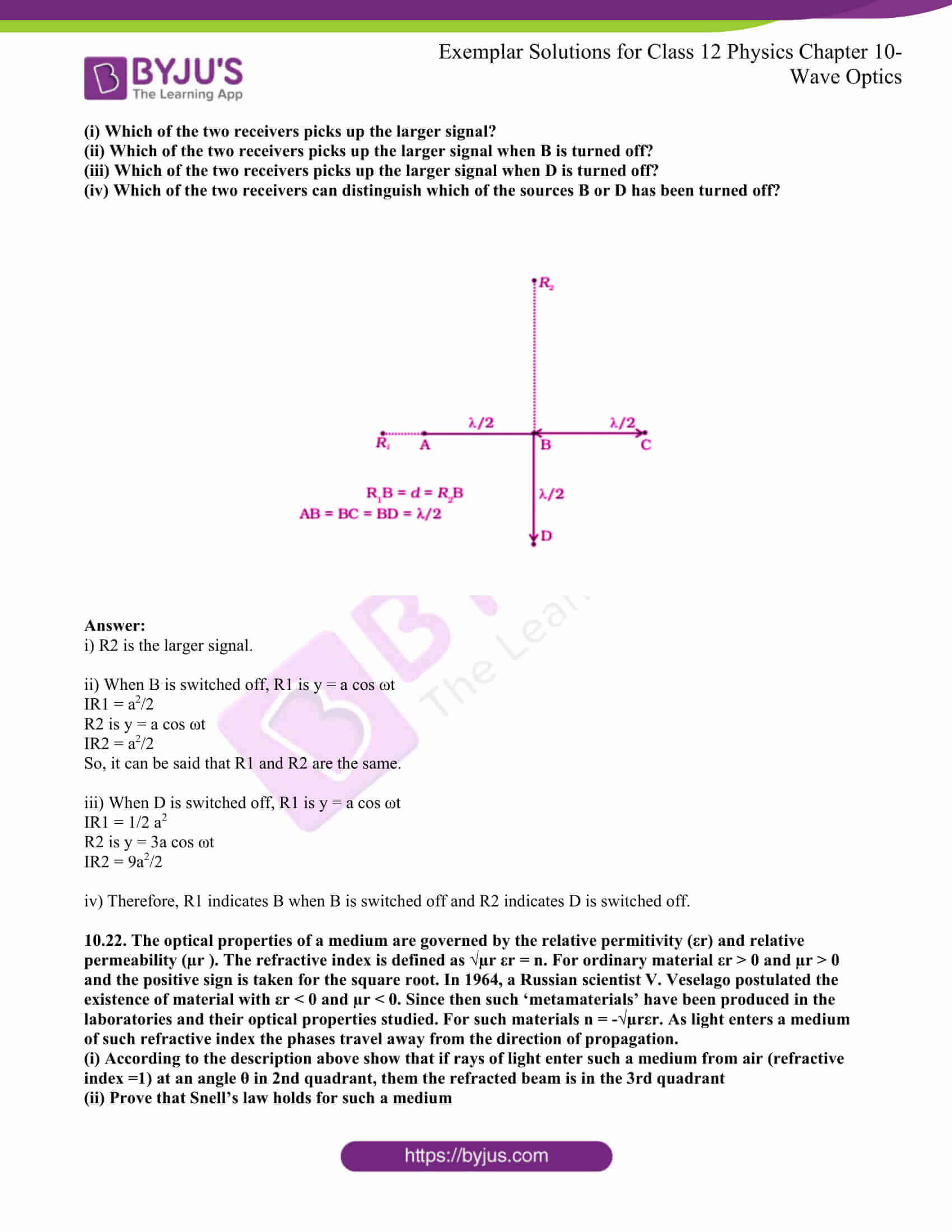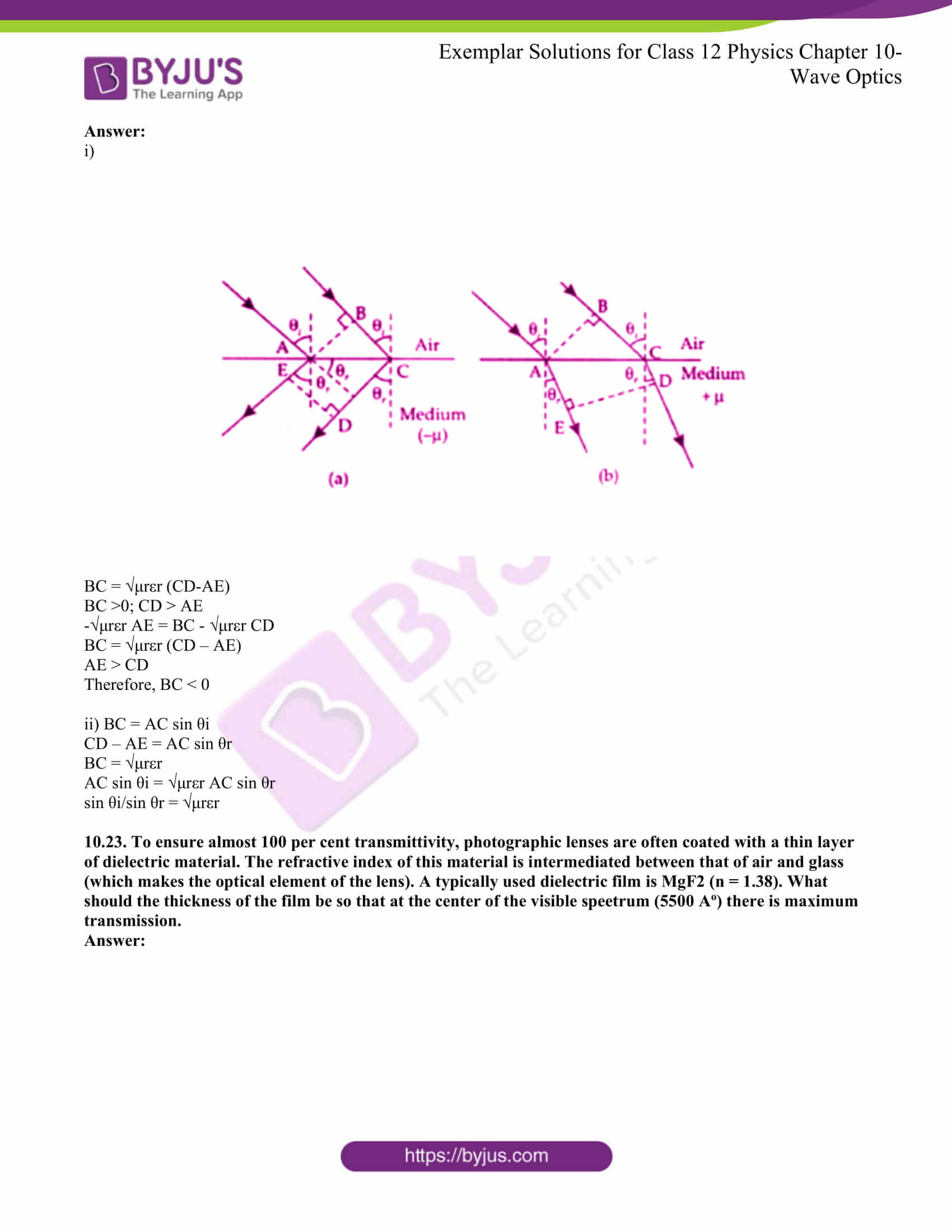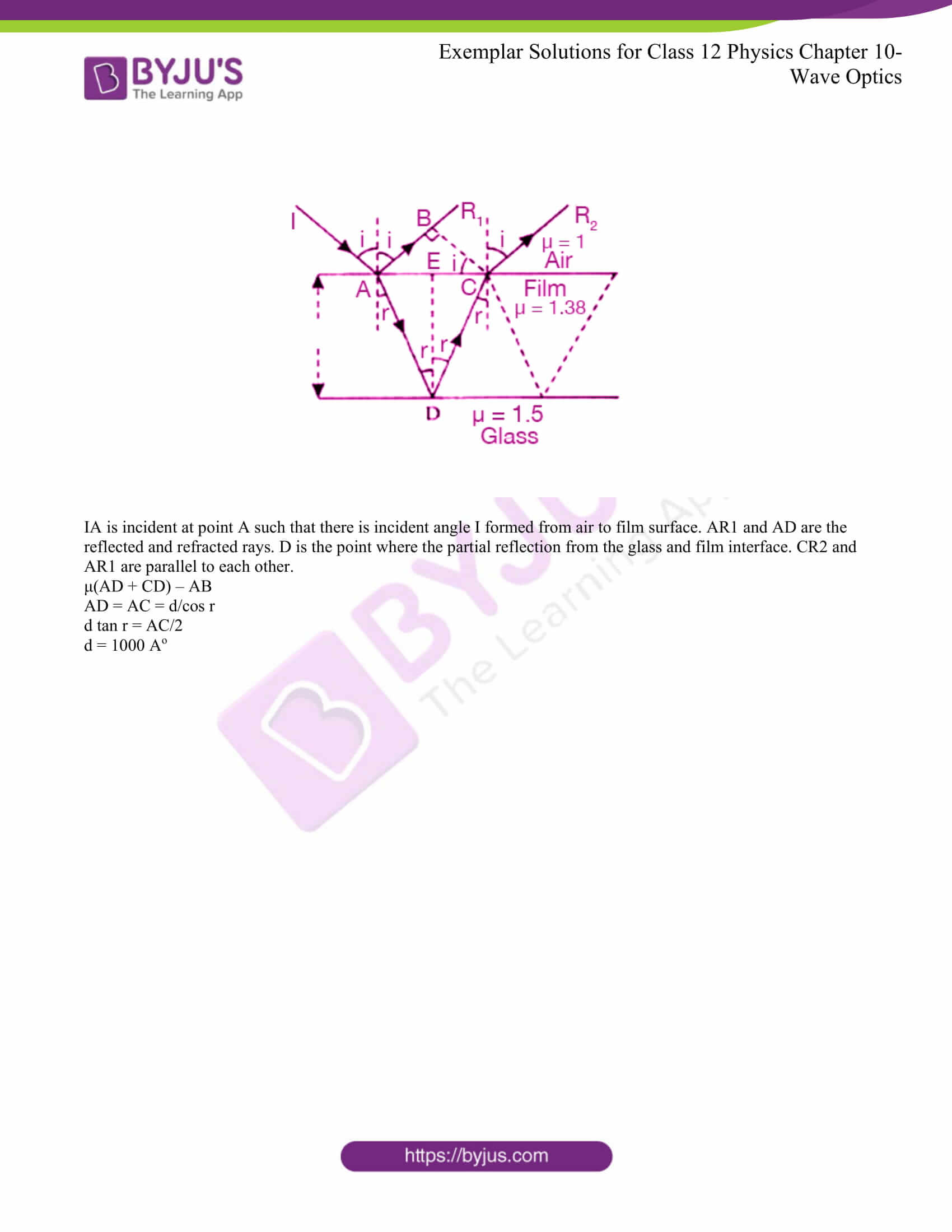### Multiple Choice Questions I

10.1. Consider a light beam incident from air to a glass slab at Brewster’s angle as shown in the figure.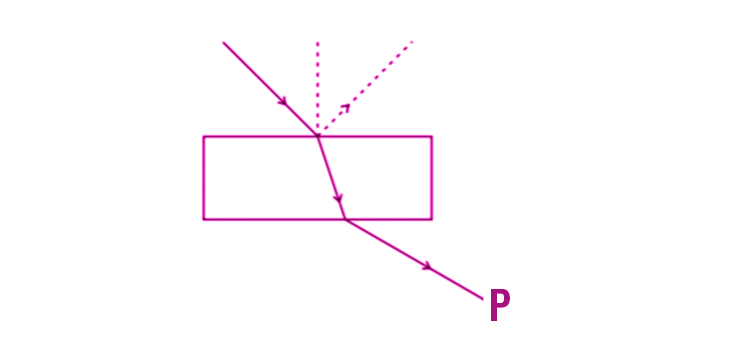A polaroid is placed in the path of the emergent ray at point P and rotated about an axis passing through the centre and perpendicular to the plane of the polaroid

(a) For a particular orientation, there shall be darkness as observed through the polaroid

(b) The intensity of light as seen through the polaroid shall be independent of the rotation

(c) The intensity of light as seen through the Polaroid shall go through a minimum but not zero for two orientations of the polaroid

(d) The intensity of light as seen through the polaroid shall go through a minimum for four orientations of the polaroid

The correct answer is c) The intensity of light as seen through the Polaroid shall go through a minimum but not zero for two orientations of the polaroid

10.2. Consider sunlight incident on a slit of width 104 A. The image seen through the slit shall

(a) be a fine sharp slit white in colour at the centre

(b) a bright slit white at the centre diffusing to zero intensities at the edges

(c) a bright slit white at the centre diffusing to regions of different colours

(d) only be a diffused slit white in colour

The correct answer is (a) be a fine sharp slit white in colour at the centre

10.3. Consider a ray of light incident from air onto a slab of glass (refractive index n) of width d, at an angle θ. The phase difference between the ray reflected by the top surface of the glass and the bottom surface is

(a)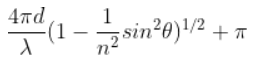(b)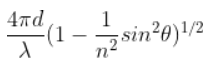(c)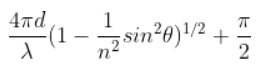(d)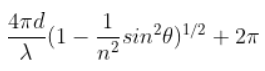10.4. In a Young’s double-slit experiment, the source is white light. One of the holes is covered by a red filter and another by a blue filter. In this case

(a) there shall be alternate interference patterns of red and blue

(b) there shall be an interference pattern for red distinct from that for blue

(c) there shall be no interference fringes

(d) there shall be an interference pattern for red mixing with one for blue

The correct answer is c) there shall be no interference fringes

10.5. The figure shows a standard two-slit arrangement with slits S1, S2. P1, P2 are the two minima points on either side of P. At P2 on the screen, there is a hole and behind P2 is a second 2- slit arrangement with slits S3, S4 and a second screen behind them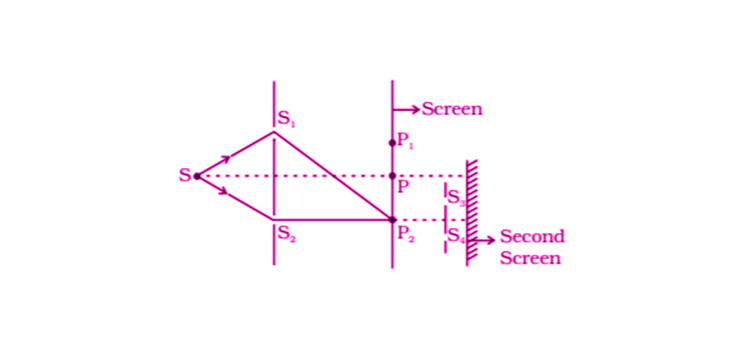(a) There would be no interference pattern on the second screen but it would be lighted

(b) The second screen would be totally dark

(c) There would be a single bright point on the second screen

(d) There would be a regular two-slit pattern on the second screen

The correct answer is d) there would be a regular two-slit pattern on the second screen

### Multiple Choice Questions II

10.6. Two sources S1 and S2 of intensity I 1 and I 2 are placed in front of a screen in the figure (a). The pattern of intensity distribution seen in the central portion is given by the figure (b). In this case which of the following statements are true.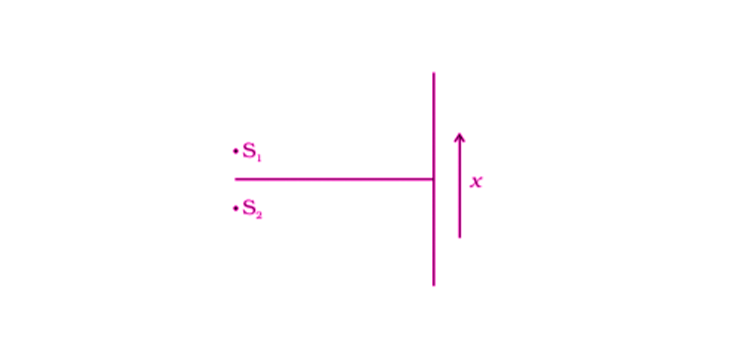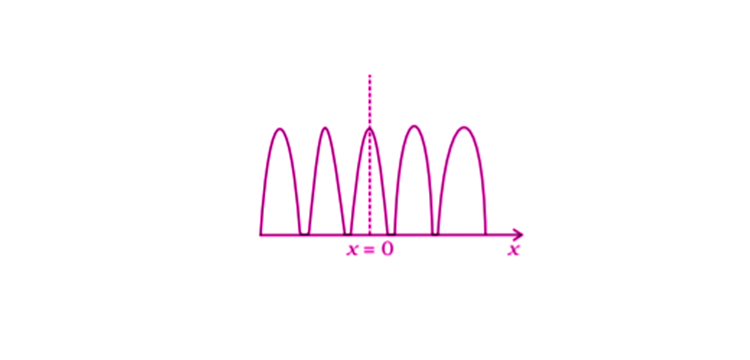(a) S1 and S2 have the same intensities

(b) S1 and S2 have a constant phase difference

(c) S1 and S2 have the same phase

(d) S1 and S2 have the same wavelength

a) S1 and S2 have the same intensities

b) S1 and S2 have a constant phase difference

c) S1 and S2 have the same phase

10.7. Consider sunlight incident on a pinhole of width 103A. The image of the pinhole seen on a screen shall be

(a) a sharp white ring

(b) different from a geometrical image

(c) a diffused central spot, white in colour

(d) diffused coloured region around a sharp central white spot

b) different from a geometrical image

d) diffused coloured region around a sharp central white spot

10.8. Consider the diffraction pattern for a small pinhole. As the size of the hole is increased

(a) the size decreases

(b) the intensity increases

(c) the size increases

(d) the intensity decreases

a) the size decreases

b) the intensity increases

10.9. For light diverging from a point source

(a) the wavefront is spherical

(b) the intensity decreases in proportion to the distance squared

(c) the wavefront is parabolic

(d) the intensity at the wavefront does not depend on the distance

a) the wavefront is spherical

b) the intensity decreases in proportion to the distance squared

10.10. Is Huygen’s principle valid for longitudinal sound waves?

Yes, Huygen’s principle is valid for longitudinal sound waves.

10.11. Consider a point at the focal point of a convergent lens. Another convergent lens of short focal length is placed on the other side. What is the nature of the wavefronts emerging from the final image?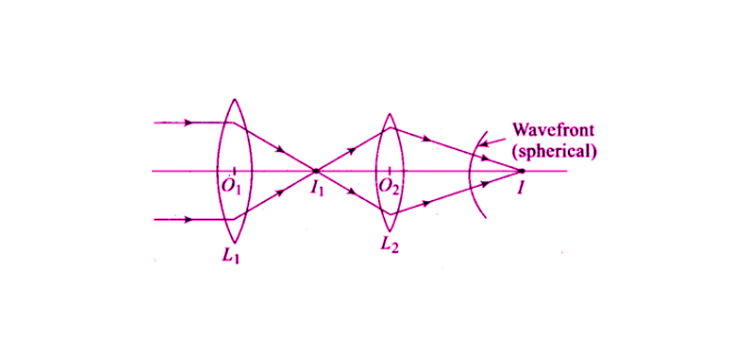The orientation of wavefront and the ray are perpendicular to each other. In the above figure, L1 is the source of parallel rays, forming I2 at the focal length of the lens. The image formed acts as L2 through which the light rays get converged into L2 and the final image I is formed.

10.12. What is the shape of the wavefront on earth for sunlight?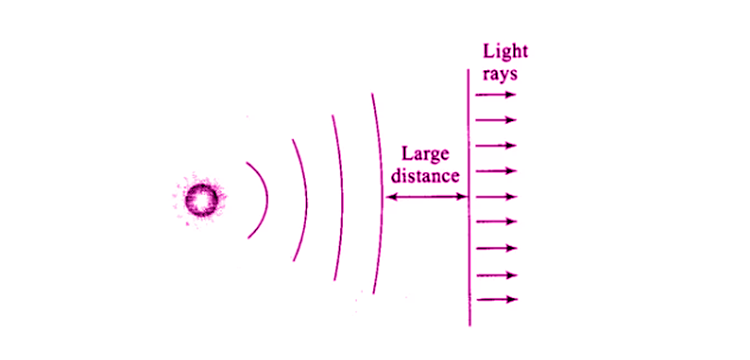We know that the distance between the sun and the earth is very large. We also know that the sun is spherical and can be considered as a point source of light which is present at a very large distance. When the point source is kept at a distance, the wavefront formed will be almost plane.

10.13. Why is the diffraction of sound waves more evident in daily experience than that of lightwave?

The frequency of sound waves varies from 20 Hz to 20,000 Hz with a wavelength of 15 m to 15 mm respectively. The diffraction of sound takes place when the size of the slit is comparable to the wavelength of the sound. The wavelength of visible light is 0.4 to 0.7 micron. The diffraction of the light doesn’t take place when the size of the slits is different from the wavelength of the visible light.

10.14. The human eye has an approximate angular resolution of φ = 5.8 × 10–4 rad and a typical photo printer prints a minimum of 300 dpi (dots per inch, 1 inch = 2.54 cm). At what minimal distance z should a printed page be held so that one does not see the individual dots.

Angular separation, φ = 5.8 × 10–4 rad

The average distance between the two dots = 2.54/300 = 0.85 × 10-2 cm

At distance z cm, the angle subtended = 0.85 × 10-2/z

Resolution angle for human = 0.85 × 10-2/z

Maximum distance between 2 dots = z = 14.5 cm

The above distance is less than the distinct vision, which normal person cannot see.

10.15. A polaroid (I) is placed in front of a monochromatic source. Another polaroid (II) is placed in front of this polaroid (I) and rotated till no light passes. A third polaroid (III) is now placed in between (I) and (II). In this case, will light emerge from (II)? Explain.

A polaroid (I) is placed in front of a monochromatic source and polaroid (II) is placed in front of polaroid (I). When the light passes through the polaroid (II), the light is unaffected.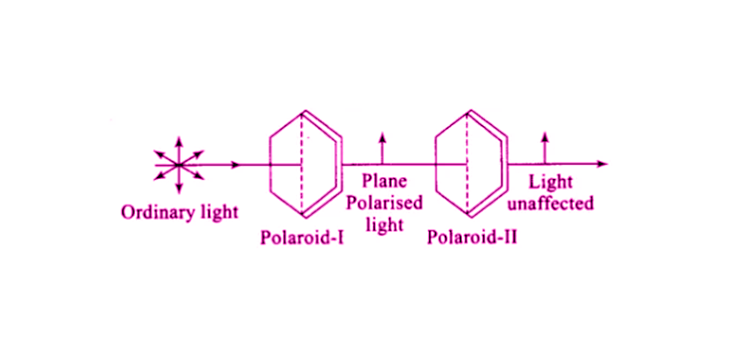Polaroid (II) is rotated such that there is no passage of light. The rotation is made such that the polaroid (II) is perpendicular to polaroid (I). When the polaroid (II) is rotated there is no passage of light.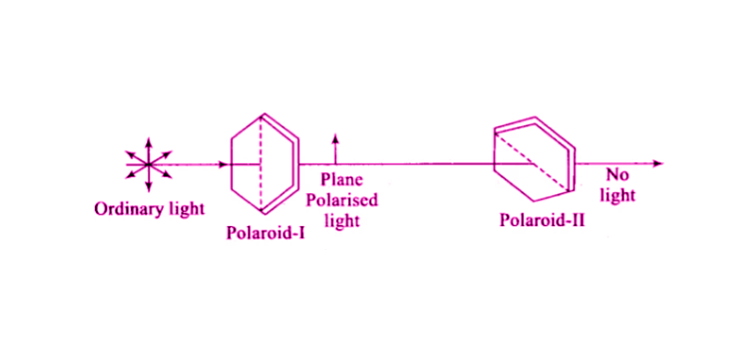When a polaroid (III) is placed between the polaroid (I) and (II) such that the polaroid (III) is not placed perpendicular to the axis, so there is no passage of light.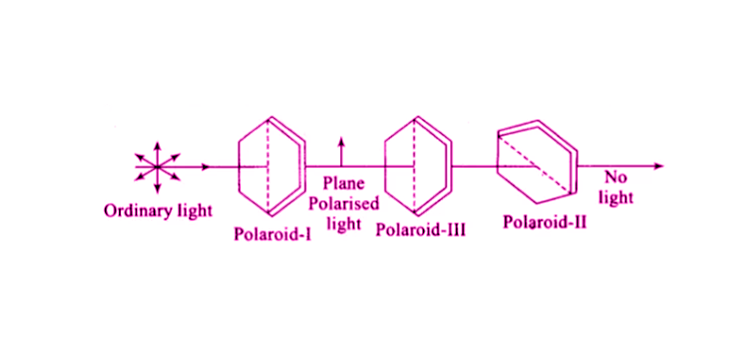10.16. Can reflection result in plane-polarized light if the light is incident on the interface from the side with higher refractive index?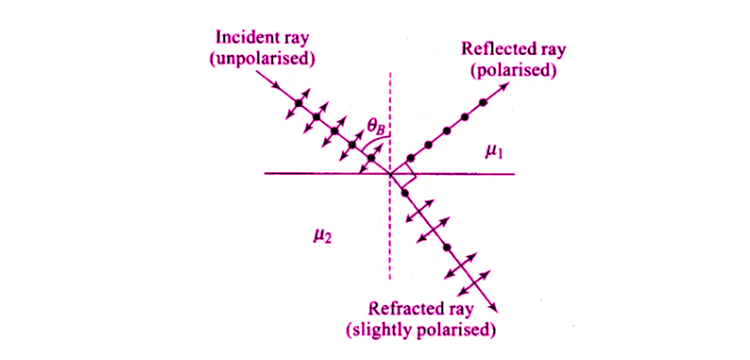When the angle of incidence is equal to the Brewster’s angle such that the transmitted light is polarized and reflected light is plane-polarized. Following is the equation: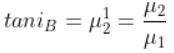Such that μ2 < μ1

When the light travels in a medium, the critical angle is given as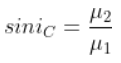Where μ2 < μ1

So, it can be said that |tan iB| > |sin iC| when iB < iC.

10.17. For the same objective, find the ratio of the least separation between two points to be distinguished by a microscope for light of 5000 Ao and electrons accelerated through 100V used as the illuminating substance.

5000 Ao = 5000 × 10-10 m

1/d = 2 sin β/1.22λ

dmin = 1.22 λ/ 2 sin β

λd = 1.22/10 × 10-10 m

When the 100V light is used, d’min = 1.22 λd/ 2 sin β

d’min = 1.22 × 1.22 × 10-10/2 sin β

The required ratio = dmin/d’min = 1.22/5000 = 0.244 × 10-3

10.18. Consider a two-slit interference arrangements such that the distance of the screen from the slits is half the distance between the slits. Obtain the value of D in terms of λ such that the first minima on the screen falls at a distance D from the centre O.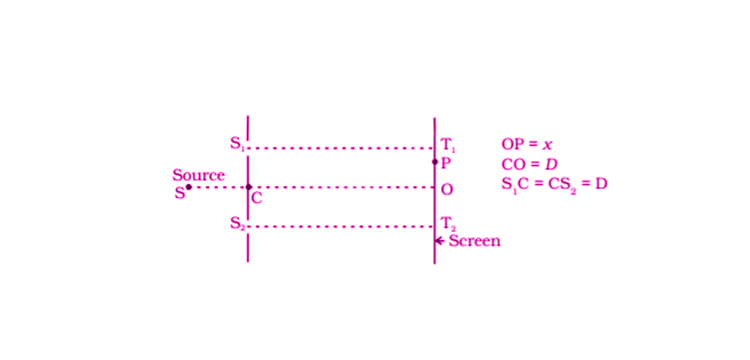The minima will occur when ∆x = S2P – S1P = (2n-1/2)λ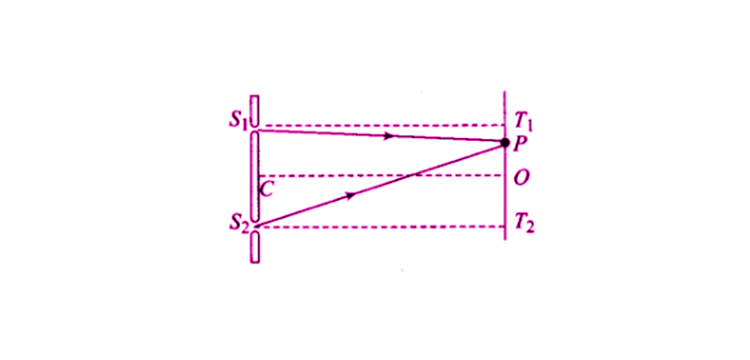S1P = √D2 + (D – x)2

S2P = √D2 + (D + x)2

T2P = D + x

T1P = D – x

[D2 + (D+x)2]-1/2 – [D2+(D-x)2]1/2 = λ/2

D = 0.404λ

10.19. The figure shown a two-slit arrangement with a source which emits unpolarised light. P is a polariser with axis whose direction is not given. If I0 is the intensity of the principal maxima when no polarizer is present, calculate in the present case, the intensity of the principal maxima as well as of the first minima.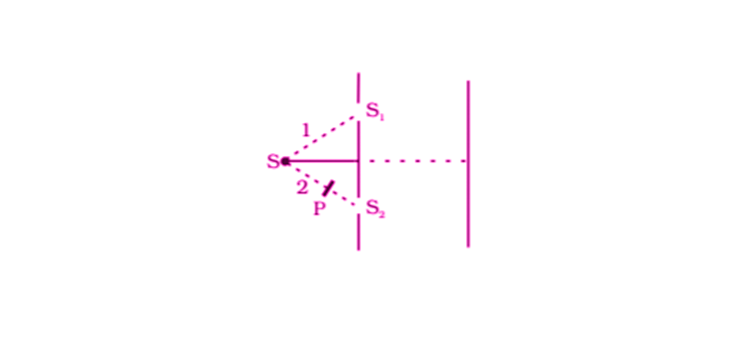Amplitude of the wave in perpendicular polarisation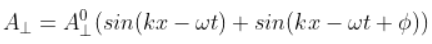Amplitude of the wave in parallel polarisation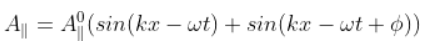Intensity at the first minima with polariser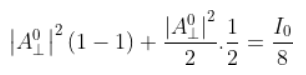10.20. AC = CO = D, S1 C = S2 C = d << D A small transparent slab containing material of µ =1.5 is placed along AS2. What will be the distance from O of the principal maxima and of the first minima on either side of the principal maxima obtained in the absence of the glass slab?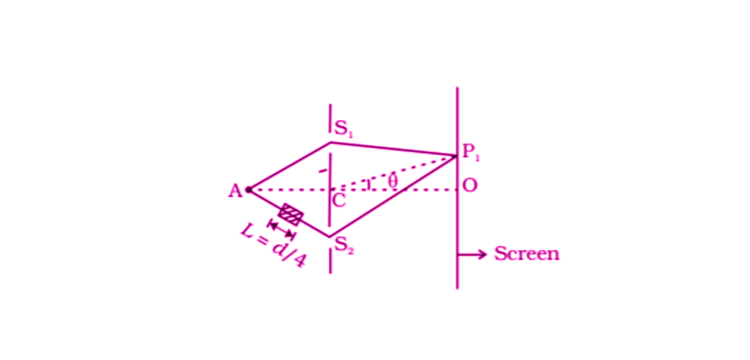∆x = 2d sin θ+(μ-1)L

sin θ0 = -1/16

From central maxima, OP = -D/16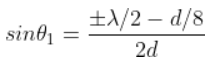On the positive side,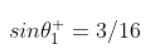On the negative side,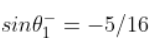The first principal maxima on the positive side is at a distance, which is above point O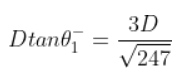The first principal minima on the negative side is at distance, which is below point O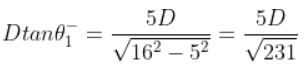10.21. Four identical monochromatic sources A, B, C, D as shown in the figure produce waves of the same wavelength λ and are coherent. Two receiver R1 and R2 are at great but equal distances from B.

(i) Which of the two receivers picks up the larger signal?

(ii) Which of the two receivers picks up the larger signal when B is turned off?

(iii) Which of the two receivers picks up the larger signal when D is turned off?

(iv) Which of the two receivers can distinguish which of the sources B or D has been turned off?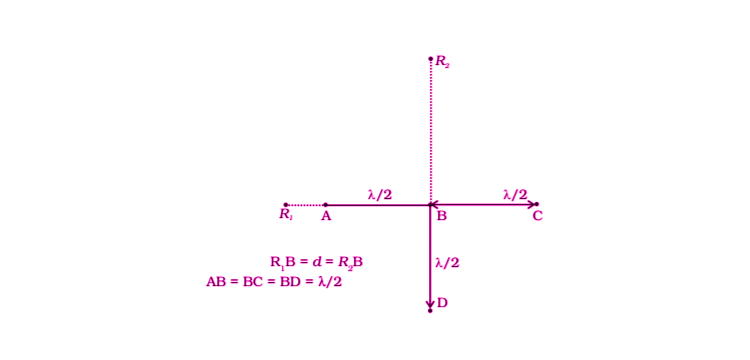i) R2 is the larger signal.

ii) When B is switched off, R1 is y = a cos ꞷt

IR1 = a2/2

R2 is y = a cos ꞷt

IR2 = a2/2

So, it can be said that R1 and R2 are the same.

iii) When D is switched off, R1 is y = a cos ꞷt

IR1 = 1/2 a2

R2 is y = 3a cos ꞷt

IR2 = 9a2/2

iv) Therefore, R1 indicates B when B is switched off and R2 indicates D is switched off.

10.22. The optical properties of a medium are governed by the relative permittivity (εr) and relative permeability (µr ). The refractive index is defined as √µr εr = n. For ordinary material εr > 0 and µr > 0 and the positive sign is taken for the square root. In 1964, a Russian scientist V. Veselago postulated the existence of material with εr < 0 and µr < 0. Since then such ‘metamaterials’ have been produced in the laboratories and their optical properties studied. For such materials n = -√µrεr. As light enters a medium of such refractive index the phases travel away from the direction of propagation.

(i) According to the description above show that if rays of light enter such a medium from the air (refractive index =1) at an angle θ in 2nd quadrant, them the refracted beam is in the 3rd quadrant

(ii) Prove that Snell’s law holds for such a medium

i)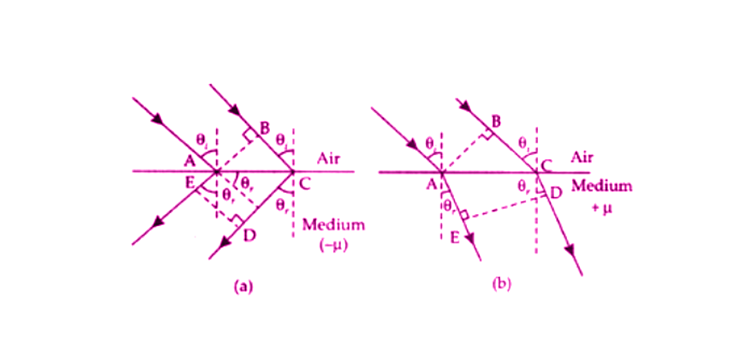BC = √μrεr (CD-AE)

BC >0; CD > AE

-√μrεr AE = BC – √μrεr CD

BC = √μrεr (CD – AE)

AE > CD

Therefore, BC < 0

ii) BC = AC sin θi

CD – AE = AC sin θr

BC = √μrεr

AC sin θi = √μrεr AC sin θr

sin θi/sin θr = √μrεr

10.23. To ensure almost 100 per cent transmissivity, photographic lenses are often coated with a thin layer of dielectric material. The refractive index of this material is intermediated between that of air and glass (which makes the optical element of the lens). A typically used dielectric film is MgF2 (n = 1.38). What should the thickness of the film be so that at the centre of the visible spectrum (5500 Ao) there is maximum transmission?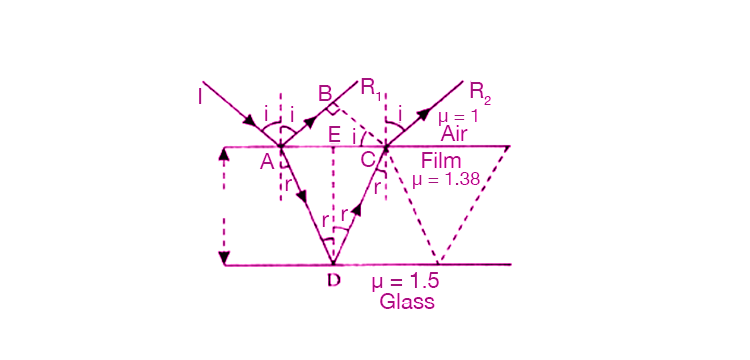IA is incident at point A such that there is an incident angle I formed from air to film surface. AR1 and AD are the reflected and refracted rays. D is the point where the partial reflection from the glass and film interface. CR2 and AR1 are parallel to each other.

AD = AC = d/cos r

d tan r = AC/2

d = 1000 Ao

Wave optics is the physical branch of optics used in different scientific studies. Different phenomena that occur are polarisation, interference and diffraction. With the help of a mirror, lens or aperture, the scattering of light can be measured.

Here are some of the fields where wave optics are utilised.

1. Electrical Engineering
2. Optical Engineering
3. Applied Physics

### Concepts Discussed in the NCERT Class 12 Physics Exemplar for Chapter 10 Wave Optics

1. Introduction
2. Huygens Principle – Statement and Experiment
3. Refraction and Reflection of Plane Waves Using Huygens Principle
4. Refraction of a Plane Wave
5. Refraction at a Rarer Medium
6. Reflection of a Plane Wave by a Plane Surface
7. The Doppler Effect – Statement, Formula and Significance
8. Coherent and Incoherent Addition of Waves – Interference Pattern Studies and Formula Derivation
9. Interference of Light Waves and Young’s Experiment – Statement, Formula Derivation, Numerical Problems and Significance
10. Diffraction
11. The Single Slit
12. Seeing the Single Slit Diffraction Pattern
13. Resolving Power of Optical Instruments
14. The Validity of Ray
15. Polarisation
16. Polarisation by Scattering
17. Polarisation by Reflection

Also Access

NCERT Solutions for Class 12 Maths Chapter 10

CBSE Notes for Class 12 Maths Chapter 10

### Why Opt for BYJU’S

BYJU’S subject experts provide the guidelines necessary for students to score good marks in Class 12 and entrance examinations. Along with study materials, BYJU’S subject experts provide learners with timely feedback after each assignment.